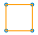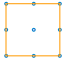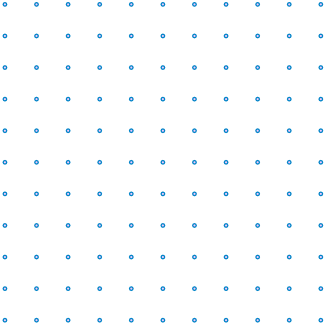# Activity: Drawing Squares

For this activity all you need is a grid of dots, a pencil and your brain.

### Let us discover how many squares you can make on different grids:Note: "1 by 1" means how many sides (not how many dots).

### 1 by 1

 Well, that's easy, there's just one:### 2 by 2

 That seems to be easy too. There are four of them, aren't there?But wait, that's not the complete answer. There's also this bigger one:That makes five squares altogether - four 1 by 1 squares and one 2 by 2 square

### 3 by 3

 Over to you now. Here's the grid:Hint: For the 3 by 3 case, you will expect to get 1 by 1 squares, 2 by 2 squares and 3 by 3 squares. How many of each?Now you can start to fill in a table:

 How Many 1 by 1 squares How Many 2 by 2 squares How Many 3 by 3 squares How Many 4 by 4 squares How Many 5 by 5 squares Total 1 by 1 Grid: 1 1 2 by 2 Grid: 4 1 5 3 by 3 Grid: 4 by 4 Grid: 5 by 5 Grid:

## Did you notice anything about the numbers in the table?

They are all square numbers:

• 12 = 1,
• 22 = 4,
• 32 = 9,
• etc ...

and the totals are found by adding together square numbers.

### Formula to The Rescue ... !

There is actually a formula for adding the first n square numbers:

Sn = n(n+1)(2n+1) / 6

### Example: The number of squares in the 5 by 5 case

Try substituting n = 5 into the formula:

Sn = n(n+1)(2n+1) / 6
S5 = 5 × (5+1) × (2×5+1) / 6
S5 = 5 × 6 × 11 / 6
S5 = 55

## So, we seem to have solved the question. Yipee!

### But wait ... there's more!

I said you would need to use your brains. Let's go back to the 2 by 2 case:

### 2 by 2

There is another square too, this one:Why is it a square? It has four equal sides and four right angles, so that's a square.

So, that makes six squares altogether.

Four 1 by 1 squares, one 2 by 2 square and one x by x square.

What is the value of x? We can use Pythagoras' Theorem to find it:

x2 = 12 + 12 = 1 + 1 = 2
So x =  √2

So, we have four 1 by 1 squares, one 2 by 2 square and one √2 by √2 square.

### 3 by 3

• Are there any more squares?

YES! Can you find them?

### 4 by 4 and 5 by 5

Also try the 4 by 4 grid, and the 5 by 5 grid

As you proceed, you will find squares like these:## What are the lengths of the sides of these squares?

You can use Pythagoras' Theorem to work that out yourself

### In each case, how many do you get of each one?

 How Many 1 by 1 How Many 2 by 2 How Many 3 by 3 How Many 4 by 4 How Many 5 by 5 How Many √2 by  √2 How Many √5 by √5 How Many √8 by √8 How Many √10 by √10 How Many √13 by √13 How Many √17 by √17 Total 1 by 1 Grid: 1 1 2 by 2 Grid: 4 1 1 6 3 by 3 Grid: 4 by 4 Grid: 5 by 5 Grid: